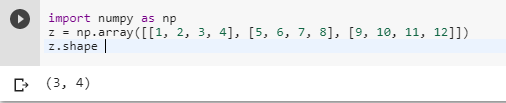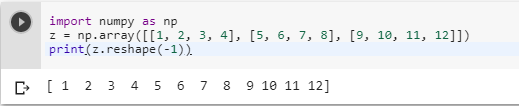2 views
in Python

A numpy matrix can be reshaped into a vector using reshape function with parameter -1. But I don't know what -1 means here.

For example:

a = numpy.matrix([[1, 2, 3, 4], [5, 6, 7, 8]])

b = numpy.reshape(a, -1)

The result of b is: matrix([[1, 2, 3, 4, 5, 6, 7, 8]])

Does anyone know what -1 means here? And it seems python assign -1 several meaning, such as array[-1] means the last element. Can you give an explanation?

by (106k points)
edited by

The reason why we use the -1 is that it provides the new shape to the existing dimension. Also, the new shape should be compatible with the original shape.

Let’s see how the numpy allow us to give one of the new shape parameters as -1.

For example : (2,-1). After seeing the shape the numpy will look at the length of the array and remaining dimensions and it will make sure it satisfies the above-mentioned criteria.

Below is an example that shows how it is done:-

import numpy as np

z = np.array([[1, 2, 3, 4], [5, 6, 7, 8], [9, 10, 11, 12]])

z.shapeNow the real thing comes as you will trying to reshape with (-1). This will result in new shape which will be (12) and this new shape is compatible with original shape (3,4).

import numpy as np

z = np.array([[1, 2, 3, 4], [5, 6, 7, 8], [9, 10, 11, 12]])

print(z.reshape(-1))